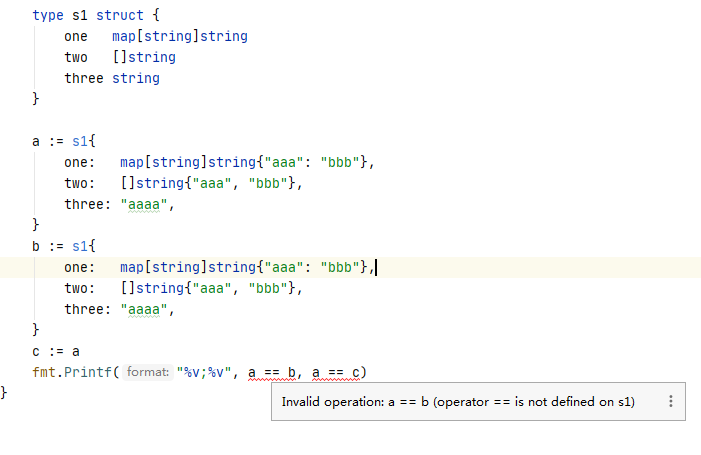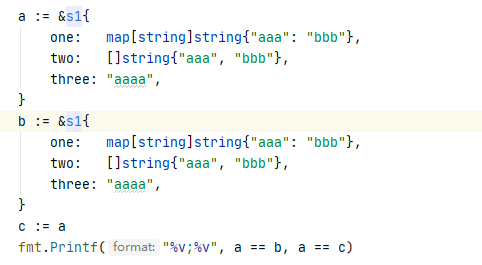# [Go] Can structure/structure pointer be compared with operator == is not defined error

Can two instances of the same struct be compared == !=

Can two different instances of struct be compared == !=

If all the member variables of the structure are comparable, then the structure can be compared.
If there are incomparable member variables in the structure, then the structure cannot be compared

```    type s2 struct {
name string
}
aa: = s2{
name: " aa " ,
}
bb: = s2{
name: " aa " ,
}
fmt.Printf( " %v\n " , aa == bb)```

This returns true

If it is a structure pointer, return false

When there are incomparable fields, an error will be reported at compile timeChange to a structure pointer, there will be no errorReturn result false; true

Code:

```    type s1 struct {
one map[ string ] string
two [] string
three string
}

a: = & s1{
one: map[ string ] string { " aaa " : " bbb " },
two: [] string { " aaa " , " bbb " },
three: " aaaa " ,
}
b: = & s1{
one: map[ string ] string { " aaa " : " bbb " },
two: [] string { " aaa " , " bbb " },
three: " aaaa " ,
}
c : = a
fmt.Printf( " %v;%v " , a == b, a == c)```